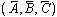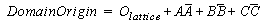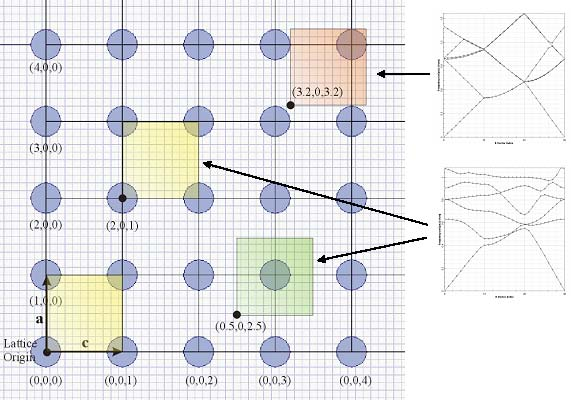In case the simulated unit cell or supercell structure has inversion symmetry the

operator matrix in the eigenvalue problem becomes a real symmetric matrix. This can be used to achieve significant savings in storage and as well as speed improvement. The inversion symmetry in the design is not detected automatically but user can notify the solver about its presence by checking the Inversion Symmetry check box. To obtain correct results,however, the unit cell (or supercell) has to have the inversion symmetry around the Domain Origin. The Domain Origin is specified in terms of  the original lattice vectorsand the lattice origin : OlatticeIt has great importance when one wants to use inversion symmetry option as well when a defect supercell is simulated. Figure 3 below shows the simulation domain of a unit cell for Domain Origin (2,0,1) and (0.5,0,2.5). Notice that the refractive index for the simulation is obtained from different parts of the layout. For the current sample we can leave the domain origin as (0,0,0) knowing that the unit cell has inversion symmetry. Check the Inversion Symmetry check box and run the simulation again (either TE, TM or both). Notice the simulation time improvement. You can deduce the simulation time from the Notification tab messages. What is going to happen if the simulation domain does not have an inversion symmetry? In such case the results will be incorrect. The solver simply samples the refractive index only on half of the domain assuming the second half is defined by the inversion symmetry. Set the Domain Origin to (3.2,0,3.2). Clearly Domain selected does not have an inversion symmetry (see figure below) and the results are incorrect. On the other hand the Domain Origin can be selected as (0.5,0,2.5) and possess the inversion symmetry again (green unit cell).Figure 3: Inversion Symmetry and Domain Origin

It should be now clear that for simulations of unit cell it would be sufficient to have
lattice structure of dimensions 1×1. The Domain Origin becomes also important in
defining the supercells containing defects as for example a PC fiber or linear defects
(waveguides).